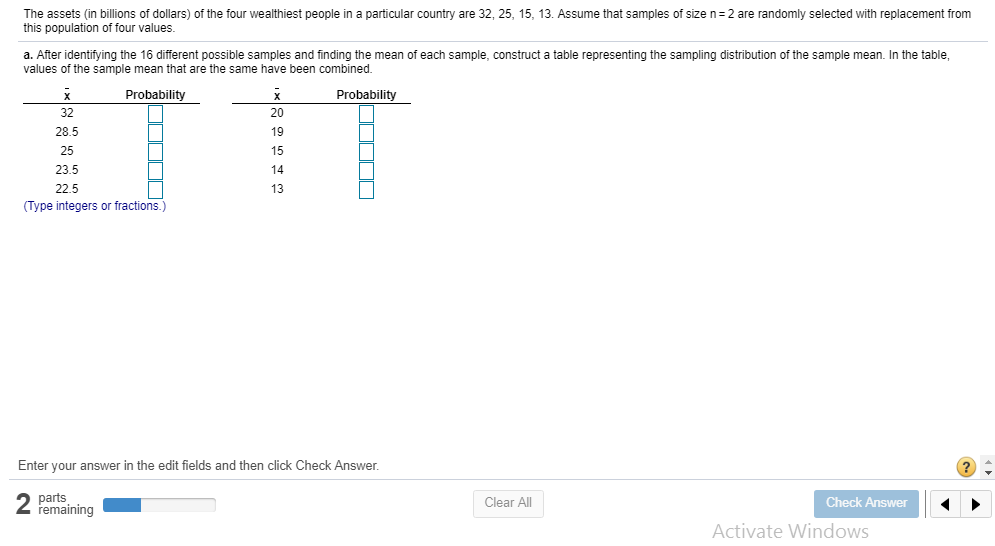# The assets (in billions of dollars) of the four wealthiest people in a particular country are 32, 25, 15, 13. Assume that samples of size n= 2 are randomly selected with replacement from this population of four values. a. After identifying the 16 different possible samples and finding the mean of each sample, construct a table representing the sampling distribution of the sample mean. In the table, values of the sample mean that are the same have been combined. Probability Probability 32 28.5 25 23.5 22.5 20 19 15 14 13 (Type integers or fractions.) Enter your answer in the edit fields and then click Check Answer. parts remaining Clear All Check Answer Activate Windows

Questionhelp_outlineImage TranscriptioncloseThe assets (in billions of dollars) of the four wealthiest people in a particular country are 32, 25, 15, 13. Assume that samples of size n= 2 are randomly selected with replacement from this population of four values. a. After identifying the 16 different possible samples and finding the mean of each sample, construct a table representing the sampling distribution of the sample mean. In the table, values of the sample mean that are the same have been combined. Probability Probability 32 28.5 25 23.5 22.5 20 19 15 14 13 (Type integers or fractions.) Enter your answer in the edit fields and then click Check Answer. parts remaining Clear All Check Answer Activate Windows fullscreen

3 Ratings

### Want to see this answer and more?

Experts are waiting 24/7 to provide step-by-step solutions in as fast as 30 minutes!*